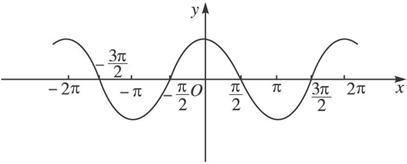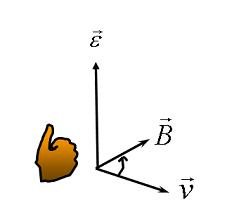搜索

# 向量点乘叉乘等理解和应用发表于 2020-4-15 08:25:24 | 显示全部楼层 |阅读模式

### 51Halcon诚邀您的加入，专注于机器视觉开发与应用技术，我们一直都在努力！

x
1.标量和矢量

2（1,2,3) = (2,4,6)

(2,4,6)/2=（1,2,3)

2.矢量和矢量的加减（平行四边形定则只适用于两个非零非共线向量的加减。）

A(X1,Y1) B(X2,Y2)，则A+B=（X1+X2，Y1+Y2），A-B=（X2-X1，Y2-Y1）

（1,2,3)  +(2,4,6)  = （3,6,9）

（3,6,9）-(2,4,6)=（1,2,3)

A向量-B向量 = 由B指向A 的向量

A 点-B点= B点指向A点的向量

void Start () {
player = GameObject.FindGameObjectWithTag(GameTag.player).transform;
offset = transform.position - player.position;

}

3.向量模    Vector3.magnitude4.向量点乘

，定义它们的数量积（又叫内积、点积）为以下实数：，它们的夹角为，则内积定义为以下实数：dot(a,b)              a * b = |a| * |b| * cosθcosθ 的值 在 0-90度    a * b = |a| * |b| * cosθ  >0

90度     a * b = |a| * |b| * cosθ  = 0

90-180度    a * b = |a| * |b| * cosθ < 01.图形学中表示光照强度当a 和e 都是单位向量时 a * b = |a| * |b| * cosθ  =   cosθ

float3 normalDir =  normalize(mul(v.normal,_World2Object));   //模型空间到世界空间  方向转换  矩阵--_World2object

fixed3 lightDir = normalize(_WorldSpaceLightPos0.xyz) ; // ，平行光，对顶点来说，光位置就是光方向

// LightColor0.rgb   平行光颜色   _Diffuse.rgb  自定义颜色  ， 标准化的向量点积求出 cosθ  ,

//使用 cosθ 表示光强，和法线垂直最亮，平行最暗

float3 diffuse = _LightColor0.rgb*max(dot(normalDir,lightDir),0)*_Diffuse.rgb;

2.位置判断

float c = Vector3.Dot (a, b);

5.向量叉乘

a ∧ b = |a| * |b| * sinθ

(a,b,c)×(x,y,z)=(bz-cy,cx-az,ay-bx)叉积的定义：c =a x b 其中a,b,c均为向量。即两个向量的叉积得到的还是向量！
性质1：c⊥a，c⊥b，即向量c垂直与向量a,b所在的平面。
性质2：模长|c|=|a||b|sin<a,b>
性质3：满足右手法则。从这点我们有axb ≠ bxa，而axb = – bxa。所以我们可以使用叉积的正负值来判断向量a，b的相对位置，即向量b是处于向量a的顺时针方向还是逆时针方向。

1 .计算出两个向量的夹角。
下面是示例代码:

[csharp] view plain copy

using UnityEngine;

using System.Collections;

public class MainScript : MonoBehaviour

{        //向量a

private Vector3 a;

//向量b

private Vector3 b;

void Start ()

{

//向量的初始化

a = new Vector3 (1, 2, 1);

b = new Vector3 (5, 6, 0);

}

void OnGUI ()

{

//点积的返回值

float c = Vector3.Dot (a, b);

//向量a,b的夹角,得到的值为弧度，我们将其转换为角度，便于查看！

float angle = Mathf.Acos (Vector3.Dot (a.normalized, b.normalized)) * Mathf.Rad2Deg;

GUILayout.Label ("向量a，b的点积为：" + c);

GUILayout.Label ("向量a，b的夹角为：" + angle);

//叉积的返回值

Vector3 e = Vector3.Cross (a, b);

Vector3 d = Vector3.Cross (b, a);

//向量a,b的夹角,得到的值为弧度，我们将其转换为角度，便于查看！

angle = Mathf.Asin (Vector3.Distance (Vector3.zero, Vector3.Cross (a.normalized, b.normalized))) * Mathf.Rad2Deg;

GUILayout.Label ("向量axb为：" + e);

GUILayout.Label ("向量bxa为：" + d);

GUILayout.Label ("向量a，b的夹角为：" + angle);

}

}

上面的示例中，我们定义了两个向量a和b。分别求出了他们的点积和叉积，并通过点积和叉积来反过来计算他们的夹角。

这里要说明的是:
通过叉积计算度数是通过公式|c|=|a||b|sin<a,b>来逆向求值。|c| 其实就是叉积的模，换句话说，也代表着Vector3.Distance (Vector3.zero, Vector3.Cross (a.normalized, b.normalized))的值。

2.用来判断一个向量在另一个向量的左侧还是右侧，通过叉乘后向量的方向判断向量的位置

float GetAngularSpeed()
{
// 求出夹角
float angle = Vector3.Angle(transform.forward, nav.desiredVelocity);

// 求弧度
float result = angle * Mathf.Deg2Rad;
// 期望速度在机器人左侧则弧度为负，否则为正
Vector3 normal = Vector3.Cross(transform.forward, nav.desiredVelocity);
if (normal.y < 0)
{
// 说明在左侧 (A 为Z方向时，B在A的逆时针180度内，此时点积小于0，方向向下，y值小于0 )
result *= -1;
}
//if (nav.desiredVelocity == Vector3.zero)
//{
//    result = 0;
//}
return result;
}

6 . 向量求夹角------Vector3.Angle(a, b)

返回的角度是俩个向量中小于180度的角度，是不分左右的，没有负数

// 如下，控制游玩区前后左右移动    x 形区域，实现控制

// Update is called once per frame

void Update () {

{

Vector2 pos = device.GetAxis();

GameObject cameraRig = transform.parent.gameObject;

if (Vector2.Angle(Vector2.up, pos) < 45)

{

cameraRig.transform.Translate(Vector3.forward *Time.deltaTime);

}

else if(Vector2.Angle(Vector2.right, pos) < 45)

{

Debug.Log("右");

cameraRig.transform.Translate(Vector3.right *Time.deltaTime);

}

else if (Vector2.Angle(Vector2.left, pos) < 45)

{

Debug.Log("左");

cameraRig.transform.Translate(Vector3.left *Time.deltaTime);

}

else if(Vector2.Angle(Vector2.down, pos) < 45)

{

Debug.Log("下");

cameraRig.transform.Translate(Vector3.back *Time.deltaTime);

}

}

}

2.设置怪物视野

public float sightAngle = 110;
// 获取玩家
private Transform player;
// 能否看到玩家
public bool canSeePlayer = false;

bool CheckSight()
{
Vector3 dir = player.position - transform.position;
if (Vector3.Angle(transform.forward, dir) < sightAngle / 2)
{
Ray ray = new Ray(transform.position + Vector3.up * 1.7f, dir);
RaycastHit hit;
// 中间不能有障碍物
if (Physics.Raycast(ray, out hit))
{
if (hit.collider.tag == GameTag.player)
{
canSeePlayer = true;
return true;
}
}
}
return false;
}

7.向量投影 Vector3 project

a点乘b向量=a向量的模乘以b向量的模乘以cosa ，

b向量的模cosa 叫做 a向量在b向量方向上的投影，等同于点积一个单位化向量

Vector3 project = Vector3.Project(nav.desiredVelocity, transform.forward);

8.Vector3.Distance(a,b)

Vector3.Distance(a,b) 等同于(a-b).magnitude 。

• 点时，表示俩点距离，即俩个相对于原点的向量，求距离

• var other : Transform;

• if (other) {

•         var dist = Vector3.Distance(other.position, transform.position);

•         print ("Distance to other: " + dist);

• }

bool CheckVoice()
{
// 获取e玩家的脚步声
if (player.GetComponent<AudioSource>().isPlaying)
{
// 定义一条导航路径
NavMeshPath path = new NavMeshPath();
// 判断在当前的导航烘焙下，敌人是否能到达脚步声的位置
if (nav.CalculatePath(player.position, path))
{
// 定义一个拐点数组，用来存放这条路径上所有拐点位置信息(不包含起点和终点)
Vector3[] corners = path.corners;
Vector3[] pathPoints = new Vector3[corners.Length + 2];
pathPoints = transform.position;
pathPoints[pathPoints.Length - 1] = player.position;
for (int i = 0; i < corners.Length; i++)
{
pathPoints[i + 1] = corners;
}
// 此路径的总距离
float pathDistance = 0;
for (int i = 0; i < pathPoints.Length - 1; i++)
{
pathDistance += Vector3.Distance(pathPoints, pathPoints[i + 1]);    // 空间俩点间的距离
}
// 若总距离小于触发器的半径，则代表听到了声音
{
return true;
}
}
}
return false;

© 2015-2021 51Halcon机器视觉  X3.4粤ICP备15095995号粤公网安备44030602000670号